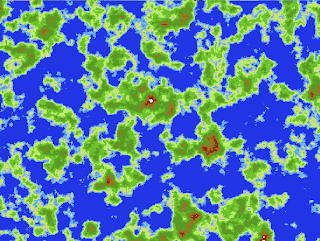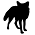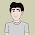## Wednesday, March 6, 2013

### Terrain Generation

Okay, time to talk about the random terrain generation algorithm, so that you too can have some cool maps!

A little research brought me to two common random terrain algorithms, Perlin Noise and Diamond-Square.  I liked the look of Diamond-Square a little more, and it seemed easier to implement than Perlin Noise anyway.  My guiding light in this part was an article from Gameprogrammer.com on fractal terrain generation.  It breaks the algorithm down into tiny bitsized steps, and is awesome!

It does, however, have some unfortunate limitations.  First and foremost, it can only build maps which have dimenions 2^n+1.  I don't like the idea of going from n=9 (513 cells) to n=10 (1025 cells) with no middle ground!  I didn't like it one bit.

Also, and perhaps even worse, while setting n to a larger number creates a larger map, it's not really that it builds a larger world... it just builds the same world at a finer and finer scale.  To get an idea of what I mean, consider a few iterations of the diamond square algorithm from the gameprogrammer article:
You don't really get any new features, you just get refinements on features already there.  Thus, whereas a grid cell in the top image may cover 10 square miles, a grid cell in the bottom image may only by 10 feet by 10 feet.  In game, then, you will have to walk over a LOT of tiles to cover much distance, which kind of sucks.  There is room for finer and finer details, but ultimately your map is limited to the features the first few steps came up with.  Especially when you are transforming it to a 2D map anyway, you will lost most of the fine details later iterations created, and you just get a HUGE boring map.

This really limits the diversity of maps you can generate, and just won't do.

To get around this, I modified the initialization step a bit.  Whereas in the original algorithm you just initialize the four corners, either all to the same value (boring) or to some random value (a little less boring), I modified it to allow you to initialize an arbitrary sized grid.The standard algorithm lets you initialize 4 corners, then works its way inward.Modified algorithm lets you initialize a grid, and works its way in through each of the regions, which all share borders and can see into their neighbors when appropriate.
Now there is a tradeoff here!  If you initialize too many points in your gird, you end up with maps which don't have much coherent structure:The tectonic forces that gave rise to this geography were a little drunk at the time...
If you have too few grid points, and rely on making decent size maps by increasing n, you get too boring of mapsI know it's more realistic... but WHYYYYY do I have to cross 12,000 tiles just to make it across the mountain range on the bottom right?
You need to tweak things to strike a balance you like.  Many things about this algorithm are customizable.Boy, doesn't that look fun!  I can't wait to spend my money to support the people who made this game!
Here's the rough idea of how to use the Diamond Square (also known as midpoint displacement) algorithm to make our 2D map:
• Generate the fractal terrain (note, this is really a 3D terrain being created)
• Normalize all the heights to lie between 0 and 1 (inclusive)
• Have a set of threshold parameters such that all points below the DeepWaterThreshold become deep water, otherwise if they are between that threshold and the ShalowWaterThreshold, they become shallow water, etc...
• Smile at your pretty map
It's not the best, there are lots of ways it could be improved.  For instance, instead of having strict thresholds, perhaps the height generated by the algorithm sets the probability that certain terrains might be picked.  That way, instead of going from solid grassland to solid darker green grassland to solid forest, there could be smoother transitions and more engaging maps.  But this is a start.

Without further adieu, here is my MidpointDisplacement.java (you could call it DiamondSquare.java):
```package com.gamexyz.utils;

public class MidpointDisplacement {
public float deepWaterThreshold,
shallowWaterThreshold,
desertThreshold,
plainsThreshold,
grasslandThreshold,
forestThreshold,
hillsThreshold,
mountainsThreshold;

public int n;
public int wmult, hmult;

public float smoothness;

public MidpointDisplacement() {

// the thresholds which determine cutoffs for different terrain types
deepWaterThreshold = 0.5f;
shallowWaterThreshold = 0.55f;
desertThreshold = 0.58f;
plainsThreshold = 0.62f;
grasslandThreshold = 0.7f;
forestThreshold = 0.8f;
hillsThreshold = 0.88f;
mountainsThreshold = 0.95f;

// n partly controls the size of the map, but mostly controls the level of detail available
n = 7;

// wmult and hmult are the width and height multipliers.  They set how separate regions there are
wmult=6;
hmult=4;

// Smoothness controls how smooth the resultant terain is.  Higher = more smooth
smoothness = 2f;
}

public int[][] getMap() {

// get the dimensions of the map
int power = MyMath.pow(2,n);
int width = wmult*power + 1;
int height = hmult*power + 1;

// initialize arrays to hold values
float[][] map = new float[width][height];
int[][] returnMap = new int[width][height];

int step = power/2;
float sum;
int count;

// h determines the fineness of the scale it is working on.  After every step, h
// is decreased by a factor of "smoothness"
float h = 1;

// Initialize the grid points
for (int i=0; i<width; i+=2*step) {
for (int j=0; j<height; j+=2*step) {
map[i][j] = MathUtils.random(2*h);
}
}

// Do the rest of the magic
while (step > 0) {
// Diamond step
for (int x = step; x < width; x+=2*step) {
for (int y = step; y < height; y+=2*step) {
sum = map[x-step][y-step] + //down-left
map[x-step][y+step] + //up-left
map[x+step][y-step] + //down-right
map[x+step][y+step];  //up-right
map[x][y] = sum/4 + MathUtils.random(-h,h);
}
}

// Square step
for (int x = 0; x < width; x+=step) {
for (int y = step*(1-(x/step)%2); y<height; y+=2*step) {
sum = 0;
count = 0;
if (x-step >= 0) {
sum+=map[x-step][y];
count++;
}
if (x+step < width) {
sum+=map[x+step][y];
count++;
}
if (y-step >= 0) {
sum+=map[x][y-step];
count++;
}
if (y+step < height) {
sum+=map[x][y+step];
count++;
}
if (count > 0) map[x][y] = sum/count + MathUtils.random(-h,h);
else map[x][y] = 0;
}

}
h /= smoothness;
step /= 2;
}

// Normalize the map
float max = Float.MIN_VALUE;
float min = Float.MAX_VALUE;
for (float[] row : map) {
for (float d : row) {
if (d > max) max = d;
if (d < min) min = d;
}
}

// Use the thresholds to fill in the return map
for(int row = 0; row < map.length; row++){
for(int col = 0; col < map[row].length; col++){
map[row][col] = (map[row][col]-min)/(max-min);
if (map[row][col] < deepWaterThreshold) returnMap[row][col] = 0;
else if (map[row][col] < shallowWaterThreshold) returnMap[row][col] = 1;
else if (map[row][col] < desertThreshold) returnMap[row][col] = 2;
else if (map[row][col] < plainsThreshold) returnMap[row][col] = 3;
else if (map[row][col] < grasslandThreshold) returnMap[row][col] = 4;
else if (map[row][col] < forestThreshold) returnMap[row][col] = 5;
else if (map[row][col] < hillsThreshold) returnMap[row][col] = 6;
else if (map[row][col] < mountainsThreshold) returnMap[row][col] = 7;
else returnMap[row][col] = 8;
}
}

return returnMap;
}
}
```
int n controls the level of detail (and hence the size of your map).  int wmult and int hmult kind of control how many (mostly) independent regions there are (and hence also control the size of your map).  The thresholds all control cutoff points for the different terrain types.  I don't want to explain how the actual algorithm itself works, check out the Gameprogrammer article if you are more curious.

I also created a class HexMapGenerator.java which calls my MidpointDisplacement algorithm (and for now that's all it does, but I hope to expand to make cooler maps, maybe place towns or resources, who knows?)
```package com.gamexyz.utils;

public class HexMapGenerator {

public HexMapGenerator() {
}

public int[][] getDiamondSquare() {
MidpointDisplacement md = new MidpointDisplacement();
return md.getMap();
}
}
```

I also updated my GameMap component to load a random map (which is remarkably fast) from the HexMapGenerator
```HexMapGenerator hmg = new HexMapGenerator();
map = hmg.getDiamondSquare();
width = map.length;
height = map.length;
```
With that, you can now make some awesome, playable looking maps!  We already know how to scroll around, zoom in and out, etc.  Notice as you scroll out that FPS goes down, that's because we already implemented the frustum culling, but when we zoom out more and more tiles are in the frustum, so it runs slower.  Play around and have some fun!

You have gained 50 XP.  Progress to Level 3: 300/600

1.Do you know how to export as a 2d grid based map?

1.When you say export, what exactly do you mean? In the getMap() code, it returns a 2D array which represents a 2d grid based map. Each item in the array represents the "height" or tile-type for that cell in the grid - for instance, if returnMap = 2, it means that the cell whose grid coordinates are (23,5) is a desert tile.

The diamond-square algorithm itself makes continuous heights (more like a 3D terrain), so to make it work well in 2D I defined threshold cutoff values - for instance any cell whose value was between 0 and 0.5 was set to 0 for deep water. Anything between 0.5 and 0.55 was set to 1 for shallow water, and so on.

If this wasn't the question you were asking, could you clarify what you meant by "export as a 2d grid based map"?

2.Good info, thank you :)

3.1.